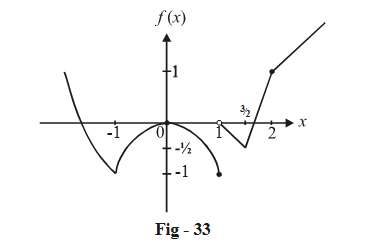# Limits Continuity Differentiability And Differentiation Set-5

Go back to  'SOLVED EXAMPLES'

Example - 12

If $$f\left( {x + y} \right) = f\left( x \right)f\left( y \right)$$ for all $$x,y \in \mathbb{R}$$ and $$f\left( x \right) = 1 + g\left( x \right)G\left( x \right)$$ ,where $$\mathop {\lim }\limits_{x \to 0} g\left( x \right) = 0\,{\rm{and }}\mathop {\lim }\limits_{x \to 0} G\left( x \right)$$   exists, prove that f (x) is continuous for all $$x \in \mathbb{R}$$ .

Solution:  We have $$\mathop {\lim }\limits_{h \to 0} g\left( h \right) = \mathop {\lim }\limits_{h \to 0} g\left( { - h} \right) = 0$$ and   $$\mathop {\lim }\limits_{h \to 0} G\left( h \right) = \mathop {\lim }\limits_{h \to 0} G\left( { - h} \right) = k\left\{ {{\rm{some\,finite\,number}}} \right\}$$

Now, LHL of f(x) (at any x)

\begin{align}& = \mathop {\lim }\limits_{h \to 0} f\left( {x - h} \right) = \mathop {\lim }\limits_{h \to 0} f\left( x \right)f\left( { - h} \right) = f\left( x \right)\mathop {\lim }\limits_{h \to 0} \left( {1 + g\left( { - h} \right)G\left( { - h} \right)} \right)\\ &= f\left( x \right)\end{align}

Similarly, RHL (at any x) of f (x) = f (x)

$$\Rightarrow f\left( x \right)$$  is continuous for all x

Example - 13

If   f\left( x \right) = \left\{ {\begin{align}{}{\left| {{x^2} - 1} \right| - 1}&{,\,\,\,\,x \le 1}\\{\left| {2x - 3} \right| - \left| {x - 2} \right|}&{,\,\,\,\,\,x > 1}\end{align}} \right\} , discuss the continuity and differentiability of f (x).

Solution:    Note that f (x) can equivalently be rewritten as:

f\left( x \right) = \left\{ {\begin{align}{}{{x^2} - 2}&\qquad\quad{x \le - 1}\\{ - {x^2}}&\qquad\quad{ - 1 < x \le 1}\\{1 - x}&\qquad\quad{1 < x \le 3/2}\\{3x - 5}&\qquad\quad{3/2 < x < 2}\\{x - 1}&\qquad\quad{2 \le x}\end{align}} \right\}

We will now simply draw the graph:We see that f (x) is non-continuous at x = 1 and non-differentiable at 4 points, x = –1, 1,  $$\frac{3}{2},2$$

Example - 14

Let a function  $$f:\mathbb{R} \to \mathbb{R}$$ be given by $$f(x + y) = f(x)f(y)$$   for all $$x,y \in \mathbb{R}$$ and  $$f(x) \ne 0$$ for any $$x \in \mathbb{R}.$$ .  If  the function $$f(x)$$   is differentiable at x = 0, show $$f'(x) = f'(0)f(x)$$ that  for all $$x \in \mathbb{R}$$ .

Solution:   Substituting x = y = 0 in the given relation, we get

$f(0) = f{(0)^2} \Rightarrow f(0) = 1\left\{ {since{\rm{ }}f\left( x \right) \ne {\rm{ }}0{\rm{ }}for{\rm{ }}\;any\;x} \right\}$

It is given that $$f(x)$$  is differentiable at x = 0, i.e., $$f'(0)$$  exists.

\begin{align}&f'(0) = \mathop {\lim }\limits_{h \to 0} \frac{{f(h) - f(0)}}{h}\\&\qquad{\rm{ }} = \mathop {\lim }\limits_{h \to 0} \frac{{f(h) - 1}}{h}{\rm{ }}...\left( i \right)\end{align}

Now we write down the expression for $$f'(x)$$  :

\begin{align}&f'(x) = \mathop {\lim }\limits_{h \to 0} \frac{{f(x + h) - f(x)}}{h}\\{\rm{ }} &\qquad= \mathop {\lim }\limits_{h \to 0} \frac{{f(x) \cdot f(h) - f(x)}}{h}\\&\qquad{\rm{ }} = f(x).\mathop {\lim }\limits_{h \to 0} \frac{{f(h) - 1}}{h}\\&\qquad{\rm{ }} = f(x) \cdot f'(0){\rm{ }}\left\{ {from{\rm{ }}\left( i \right)} \right\}\end{align}

$$\Rightarrow$$   $$f'(x) = f'(0) \cdot f(x)$$  for all

Learn from the best math teachers and top your exams

• Live one on one classroom and doubt clearing
• Practice worksheets in and after class for conceptual clarity
• Personalized curriculum to keep up with school